# Basic Geometry : How to find the length of the side of a square

## Example Questions

1 2 3 4 6 Next →

### Example Question #317 : Squares

Find the side length of a square given the area is 144.Explanation:

To solve, simply use the formula for the area of a square to solve for side length. Thus,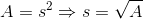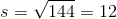### Example Question #318 : Squares

Oscar recently built his first home, with a floor plan that is both a perfect square and has an area of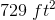. What is the length for the sides of Oscar's new home?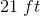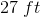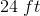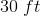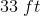Explanation:

Since both sides of a square are of equal length, we can use this formula to determine the length of both sides.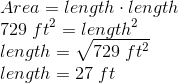### Example Question #319 : Squares

A cube has a volume of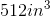. What is the length of one of the sides of one of its faces?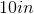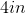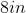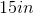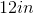Explanation:

The volume of a cube is found by multiplying the width, height, and depth. Since all sides are equal, the formula would look like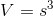. Since we know the volume, we can take the cube root of that volume to get the individual side length.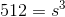What number cubed equals 512? 8 cubed gives 512.

### Example Question #320 : Squares

The diagonal of a square is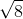centimeters. What is the length of each side?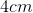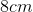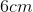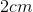Explanation:

To solve, set up an equation using the Pythagorean Theorem: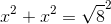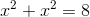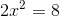divide both sides by 2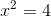take the square root of both sides### Example Question #321 : Squares

The perimeter of a square is 56cm. What is the length of the sides?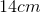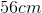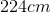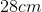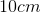Explanation:

The perimeter of a square is the sum of all the sides, or 4 times the length of one side.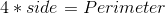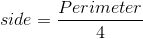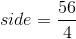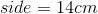### Example Question #322 : Squares

The area of a square is 36. Find the length of its sides,.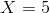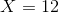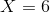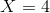Explanation:

The area of a square is given by the formula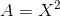,

whereis the length of all sides.

We were given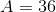,

so to findwe take the square root of both sides.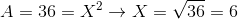.

### Example Question #323 : Squares

Know that in a Major League Baseball infield the distance between home plate and first base is 90 feet and the infield is a perfect square.

If a field designer accidentally designs an infield with an area of 3600 Square Feet, but remembers to make the infield a perfect square, what is distance between home plate and first base?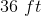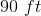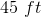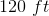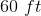Explanation:

The answer can be found by knowing that the area of 3600 square feet is smaller than the typical area of 8100 square feet.

This means the length of the square's sides must be smaller than 90 feet.

Then you can check by finding the area of the squares that would be formed by the lengths that are lower than 90 feet. 60 feet is the correct answer because 60x60=3600 Square Feet.

### Example Question #324 : Squares

True or false: A square with diagonals of length 2 has sides of length.

False

True

True

Explanation:

A square is shown below with its diagonal of length 2.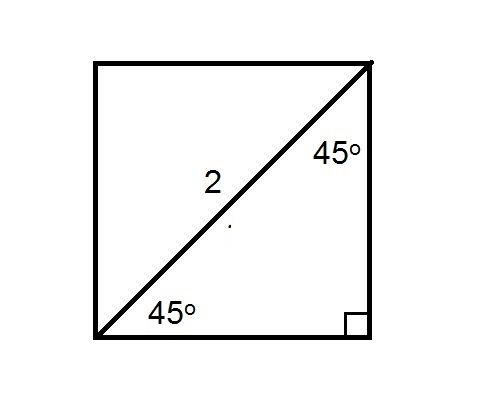Each of the triangles formed is an isosceles right triangle with congruent legs - by the 45-45-90 Triangle Theorem, they are 45-45-90 triangles. Also by the 45-45-90 Triangle Theorem, the diagonal, each leg of the triangle has length equal to the hypotenuse divided by. Therefore, the length of a leg - and a side of the square - is 2 divided by, or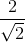.

Rationalize the denominator by multiplying both halves of the fraction by: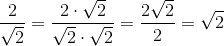### Example Question #325 : Squares

True or false: A square with area 49 has sides of length 7.

False

True

True

Explanation:

Given the length of each side of a square, the area of the square is the square of this length. Therefore, to find the sidelength, take the square root of the area. The area of the square is 49, and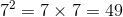, so its sidelength is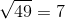.

### Example Question #326 : Squares

If the area of a square is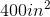, what is the measure of one of its sides?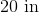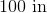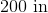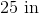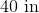Explanation:

If we are given the area of a square, in order to find the length of a side we must take the square root of the area. We need to know that the formula for area of a square is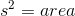(where s is side) so we plug in 400 for the area and we have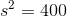To solve for s we must take the square root of both sides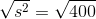and we get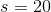When taking square roots we reject the negative if we are working with measurements. Our final answer is 20 inches.

1 2 3 4 6 Next →